# Difference between revisions of "Page theorem"

Page's theorem on the zeros of Dirichlet-functions. Let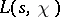be a Dirichlet-function,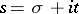, witha Dirichlet character modulo,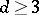. There are absolute positive constantssuch that

a)for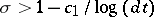,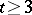;

b)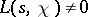for,;

c) for complexmodulo,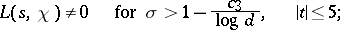(1)

d) for real primitivemodulo,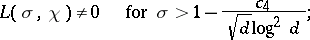(2)

e) for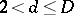there exists at most one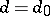,and at most one real primitive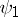modulofor which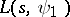can have a real zero, where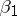is a simple zero; and for allsuch that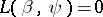,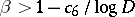with a realmodulo, one has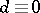().

Page's theorem on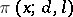, the number of prime numbers,(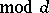) for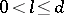, whereandare relatively prime numbers. With the symbols and conditions of Section 1, on account of a)–c) and e) one haswhere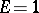orin accordance with whetherexists or not for a given; because of , for anyone has for a given,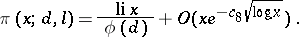(*)

This result is the only one (1983) that is effective in the sense that ifis given, then one can state numerical values of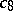and the constant appearing in the symbol. Replacement of the bound in

by the Siegel bound: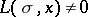for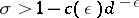,, extends the range of (*) to essentially larger,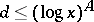for any fixed, but the effectiveness of the bound in (*) is lost, since for a givenit is impossible to estimate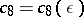and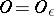.

A. Page established these theorems in .

How to Cite This Entry:
Page theorem. Encyclopedia of Mathematics. URL: http://encyclopediaofmath.org/index.php?title=Page_theorem&oldid=11725
This article was adapted from an original article by A.F. Lavrik (originator), which appeared in Encyclopedia of Mathematics - ISBN 1402006098. See original article